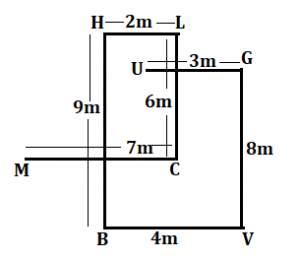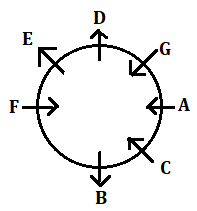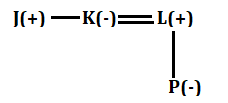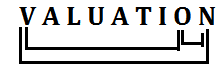Latest Banking jobs   »

# Reasoning Ability Quiz For Bank Foundation 2023- 7th January

Directions (1-2): Study the information carefully and answer the questions given below.

Point U is 3m west of point G, which is 8m north of point V. Point V is 4m east of point B. Point B is 9m south of point H. Point H is 2m west of point L. Point L is 6m north of point C. Point C is 7m east of point M.

Q1. What is the shortest distance between point U and Point M?
(a) 2√18 m
(b) √61 m
(c) √63 m
(d) 8m
(e) None of these

Q2. In which direction point C with respect to point V?
(a) North
(b) North-west
(c) North-east
(d) South-west
(e) None of these

Directions (3-5): Study the information carefully and answer the questions given below.
Seven persons A, B, C, D, E, F and G are sitting in a circular table. Some of them facing towards center and some of them facing away from center but not necessary in same order.
D sits third to the right of B. E sits immediate left of D. Two persons sit between B and G. A is immediate neighbor of C. A sits third to the right of F. Immediate neighbor of G face opposite direction to each other. G face towards the center. Neither G nor C face same direction as D. Not more than four-person face towards center.

Q3. Who among the following person sits second to the right of C?
(a) F
(b) D
(c) E
(d) G
(e) None of these

Q4. How many persons sit between A and E, when counted to the right of E?
(a) Two
(b) Three
(c) One
(d) Four
(e) None of these

Q5. How many persons face outside the center?
(a) Four
(b) Two
(c) Three
(d) Five
(e) None of these

Q6. If all the digits in the number “8154276367” arranged in ascending order from left to right then how many numbers remains same in their position?
(a) One
(b) Two
(c) Three
(d) Four
(e) None of these

Q7. If ‘A × D’ mean ‘D is the sister of A’, ‘A + D’ means ‘D is the daughter of A’, ‘A ÷ D’ means ‘A is the wife of D’, and ‘A-D’ means ‘A is brother of D’ then how will ‘K is mother of P’ be denoted?
(a) J – K ÷ P + L
(b) K + P + M × J
(c) J – K ÷ L + P
(d) K – J ÷ L + P
(e) None of these

Q8. In a row Ram sits 23rd from the left end of the row and Aman sits 25th from the right end of the row. If there are 7 people sit in between Ram and Aman then find out how many people sit in the row?
(a) 54
(b) 47
(c) 52
(d) 60
(e) Can’t be determined

Q9. How many pairs of letters are there in the word “VALUATION” each of which have as many letters between them (both forward and backward directions) in the word as they have between them in the English alphabetical series?
(a) None
(b) Two
(c) Three
(d) More than three
(e) None of these

Q10. Pointing towards photograph a person said “This girl is daughter in law of my father”. That person has no brother. How is person related to that girl?
(a) Father
(b) Father-in-law
(c) Grandfather
(d) Son
(e) None of these

Directions (11-13): Study the information carefully and answer the questions given below.

Six persons M, N, O, P, Q, and S are different weight. P is heavier than N. M is lighter than S. Q is heavier than N. M is not lighter than P and Q. S is not heaviest, and Q is not second lightest. The weight of heaviest is 70kg.

Q11. Who among the following person is third heaviest?
(a) M
(b) S
(c) P
(d) Q
(e) Can’t be determined

Q12. Who among the following is the lightest person?
(a) M
(b) N
(c) Q
(d) P
(e) None of these

Q13. If weight of M is 67kg what may be the weight of S?
(a) 60kg
(b) 63kg
(c) 61kg
(d) 69kg
(e) None of these

Q14. If in the number 39475132, 2 is multiply to each of the digit which is less than 4 and 3 is subtracted from each of the digit which is more than 4 and equal to 4 then how many digits are repeating in the number thus formed?
(a) None
(b) Four
(c) One
(d) Three
(e) Two

Q15. If all the letters in the word CONSTITUTION are arranged in alphabetical order from left to right, then how many alphabets remains same in their position?
(a) Three
(b) One
(c) None
(d) Two
(e) four

Solutions

Solutions (1-2):
Sol.S1. Ans. (b)
S2. Ans. (b)

Solution (3-5):
Sol.S3. Ans. (d)
S4. Ans. (a)
S5. Ans. (c)

S6. Ans. (b)
Sol. Original number: 8154276367
Obtained number: 1234566778

S7. Ans. (c)
Sol.S8. Ans. (e)

S9. Ans. (c)
Sol.S10. Ans. (e)
Sol. That person is husband of that girl

Solution (11-13):
Sol. O (70kg) > S > M > Q > P > N

S11. Ans. (a)
S12. Ans. (b)
S13. Ans. (d)

S14. Ans. (d)

S15. Ans. (b)
Sol. Original Word- CONSTITUTION
After operation- CIINNOOSTTTU## FAQs

### Q1. What is the shortest distance between point U and Point M? (a) 2√18 m (b) √61 m (c) √63 m (d) 8m (e) None of these

S1. Ans. (b)

#### Congratulations!